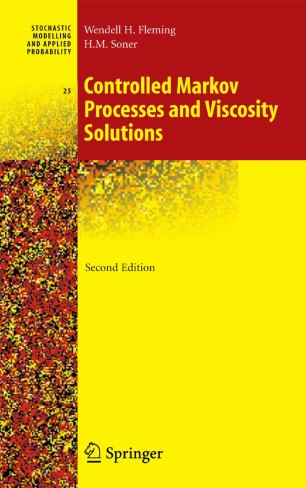# Controlled Markov Processes and Viscosity Solutions

• Wendell H. Fleming
• H.M. SonerBook

Part of the Stochastic Modelling and Applied Probability book series (SMAP, volume 25)

1. Front Matter
Pages i-xvii
2. Pages 1-55
3. Pages 57-117
4. Pages 151-198
5. Pages 199-226
6. Pages 227-259
7. Pages 261-292
8. Pages 293-320
9. Pages 321-346
10. Pages 347-373
11. Pages 375-395
12. Back Matter
Pages 397-429

### Introduction

This book is intended as an introduction to optimal stochastic control for continuous time Markov processes and to the theory of viscosity solutions. Stochastic control problems are treated using the dynamic programming approach. The authors approach stochastic control problems by the method of dynamic programming. The fundamental equation of dynamic programming is a nonlinear evolution equation for the value function. For controlled Markov diffusion processes, this becomes a nonlinear partial differential equation of second order, called a Hamilton-Jacobi-Bellman (HJB) equation. Typically, the value function is not smooth enough to satisfy the HJB equation in a classical sense. Viscosity solutions provide framework in which to study HJB equations, and to prove continuous dependence of solutions on problem data. The theory is illustrated by applications from engineering, management science, and financial economics.

In this second edition, new material on applications to mathematical finance has been added. Concise introductions to risk-sensitive control theory, nonlinear H-infinity control and differential games are also included.

Review of the earlier edition:

"This book is highly recommended to anyone who wishes to learn the dinamic principle applied to optimal stochastic control for diffusion processes. Without any doubt, this is a fine book and most likely it is going to become a classic on the area... ."

SIAM Review, 1994

### Keywords

Markov Chain Markov process Optimal control control control theory optimization programming

#### Authors and affiliations

• Wendell H. Fleming
• 1
• H.M. Soner
• 2
1. 1.Div. Applied MathematicsBrown UniversityProvidenceUSA
2. 2.Department of MathematicsCarnegie-Mellon UniversityPittsburghUSA

Industry Sectors
Pharma
Biotechnology